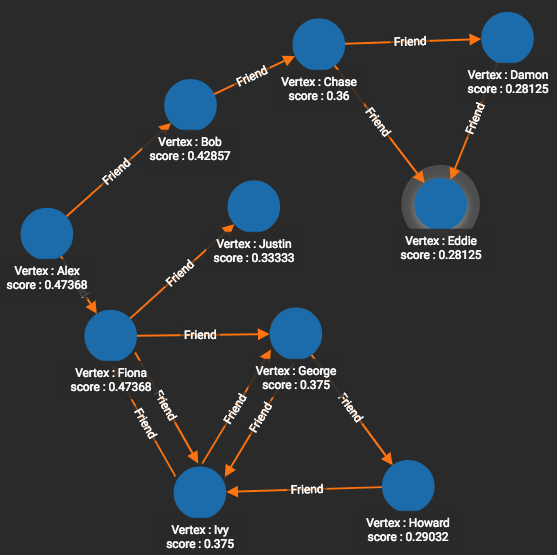# Closeness Centrality

We all have an intuitive understanding when we say a home, an office, or a store is "centrally located." Closeness Centrality provides a precise measure of how "centrally located" a vertex is. The steps below show the steps for one vertex `v`:

Step Mathematical Formula

1. Compute the average distance from vertex v to every other vertex:

$d_{avg}(v) = \sum_{u \ne v} dist(v,u)/(n-1)$

2. Invert the average distance, so we have average closeness of v:

$CC(v) = 1/d_{avg}(v)$

TigerGraph’s closeness centrality algorithm uses multi-source breadth-first search (MS-BFS) to traverse the graph and calculate the sum of a vertex’s distance to every other vertex in the graph, which vastly improves the performance of the algorithm. The algorithm’s implementation of MS-BFS is based on the paper The More the Merrier: Efficient Multi-source Graph Traversal by Then et al.

 This algorithm query employs a subquery called `cc_subquery`. Both queries are needed to run the algorithm.

## Specifications

``````tg_closeness_cent (SET<STRING> v_type, SET<STRING> e_type, INT max_hops=10,
INT top_k=100, BOOL wf = TRUE, BOOL print_accum = True, STRING result_attr = "",
STRING file_path = "", BOOL display_edges = FALSE)``````

## Parameters

Characteristic Value

Result

Computes a Closeness Centrality value (FLOAT type) for each vertex.

Required Input Parameters

• `SET<STRING> v_type`: Names of vertex types to use

• `SET<STRING> e_type`: Names of edge types to use

• `SET<STRING>` `re_type`: Names of reverse edge types to use

• `INT max_hops`: If >=0, look only this far from each vertex

• `INT top_k`: Sort the scores highest first and output only this many scores

• `BOOL wf`: If True, use Wasserman-Faust normalization for multi-component graphs

• `BOOL print_accum`: If true, output JSON to standard output

• `STRING result_attr`: If not empty, store centrality values (FLOAT) to this attribute

• `STRING file_path`: If not empty, write output to this file in CSV.

• `BOOL display_edges`: If true, include the graph’s edges in the JSON output, so that the full graph can be displayed.

Result Size

V = number of vertices

Time Complexity

O(E), E = number of edges.

Parallel processing reduces the time needed for computation.

Graph Types

Directed or Undirected edges, Unweighted edges

## Example

Closeness centrality can be measured for either directed edges (from `v` to others) or for undirected edges. Directed graphs may seem less intuitive, however, because if the distance from Alex to Bob is 1, it does not mean the distance from Bob to Alex is also 1.

For our example, we wanted to use the topology of the Likes graph, but to have undirected edges. We emulated an undirected graph by using both `Friend` and `Also_Friend` (reverse-direction) edges.

``````# Use _ for default values
RUN QUERY tg_closeness_cent(["Person"], ["Friend", "Also_Friend"], _, _,
_, _, _, _, _)``````